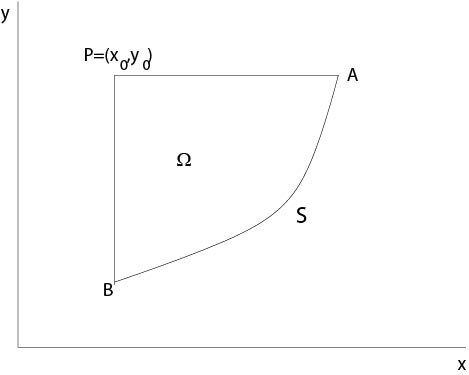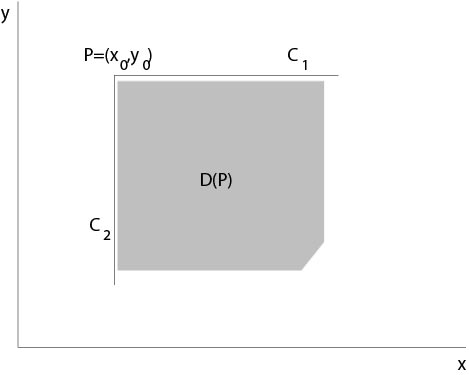$$\newcommand{\id}{\mathrm{id}}$$ $$\newcommand{\Span}{\mathrm{span}}$$ $$\newcommand{\kernel}{\mathrm{null}\,}$$ $$\newcommand{\range}{\mathrm{range}\,}$$ $$\newcommand{\RealPart}{\mathrm{Re}}$$ $$\newcommand{\ImaginaryPart}{\mathrm{Im}}$$ $$\newcommand{\Argument}{\mathrm{Arg}}$$ $$\newcommand{\norm}{\| #1 \|}$$ $$\newcommand{\inner}{\langle #1, #2 \rangle}$$ $$\newcommand{\Span}{\mathrm{span}}$$

# 4.4: A Method of Riemann

$$\newcommand{\vecs}{\overset { \rightharpoonup} {\mathbf{#1}} }$$ $$\newcommand{\vecd}{\overset{-\!-\!\rightharpoonup}{\vphantom{a}\smash {#1}}}$$$$\newcommand{\id}{\mathrm{id}}$$ $$\newcommand{\Span}{\mathrm{span}}$$ $$\newcommand{\kernel}{\mathrm{null}\,}$$ $$\newcommand{\range}{\mathrm{range}\,}$$ $$\newcommand{\RealPart}{\mathrm{Re}}$$ $$\newcommand{\ImaginaryPart}{\mathrm{Im}}$$ $$\newcommand{\Argument}{\mathrm{Arg}}$$ $$\newcommand{\norm}{\| #1 \|}$$ $$\newcommand{\inner}{\langle #1, #2 \rangle}$$ $$\newcommand{\Span}{\mathrm{span}}$$ $$\newcommand{\id}{\mathrm{id}}$$ $$\newcommand{\Span}{\mathrm{span}}$$ $$\newcommand{\kernel}{\mathrm{null}\,}$$ $$\newcommand{\range}{\mathrm{range}\,}$$ $$\newcommand{\RealPart}{\mathrm{Re}}$$ $$\newcommand{\ImaginaryPart}{\mathrm{Im}}$$ $$\newcommand{\Argument}{\mathrm{Arg}}$$ $$\newcommand{\norm}{\| #1 \|}$$ $$\newcommand{\inner}{\langle #1, #2 \rangle}$$ $$\newcommand{\Span}{\mathrm{span}}$$

Riemann's method provides a formula for the solution of the following Cauchy initial value problem for a hyperbolic equation of second order in two variables. Let

$${\mathcal S}:\ \ x=x(t), y=y(t),\ \ t_1\le t \le t_2,$$

be a regular curve in $$\mathbb{R}^2$$, that is, we assume $$x,\ y\in C^1[t_1,t_2]$$ and $$x'^2+y'^2\not=0$$. Set

$$Lu:=u_{xy}+a(x,y)u_x+b(x,y)u_y+c(x,y)u,$$

where $$a,\ b\in C^1$$ and $$c,\ f\in C$$ in a neighborhood of $${\mathcal S}$$. Consider the initial value problem

\begin{eqnarray}
\label{riem1}
Lu&=&f(x,y)\\
\label{riem2}
u_0(t)&=&u(x(t),y(t))\\
\label{riem3}
p_0(t)&=&u_x(x(t),y(t))\\
\label{riem4}
q_0(t)&=&u_y(x(t),y(t)),
\end{eqnarray}

where $$f\in C$$ in a neighbourhood of $${\mathcal S}$$ and $$u_0,\ p_0,\ q_0\in C^1$$ are given.

We assume:

1. $$u_0'(t)=p_0(t)x'(t)+q_0(t)y'(t)$$ (strip condition),
2. $${\mathcal S}$$ is not a characteristic curve. Moreover assume that the characteristic curves, which are lines here and are defined by $$x=const.$$ and $$y=const.$$, have at most one point of intersection with $${\mathcal S}$$, and such a point is not a touching point, i. e., tangents of the characteristic and $${\mathcal S}$$ are different at this point.

We recall that the characteristic equation to (\ref{riem1}) is $$\chi_x\chi_y=0$$ which is satisfied if $$\chi_x(x,y)=0$$ or $$\chi_y(x,y)=0$$. One family of characteristics associated to these first partial differential of first order is defined by $$x'(t)=1,\ y'(t)=0$$, see Chapter 2.

Assume $$u,\ v\in C^1$$ and that $$u_{xy},\ v_{xy}$$ exist and are continuous. Define the adjoint differential expression by

$$Mv=v_{xy}-(av)_x-(bv)_y+cv.$$

We have

\begin{equation}
\label{riem5}
2(vLu-uMv)=(u_xv-v_xu+2buv)_y+(u_yv-v_yu+2auv)_x.
\end{equation}

Set

\begin{eqnarray*}
P&=&-(u_xv-x_xu+2buv)\\
Q&=&u_yv-v_yu+2auv.
\end{eqnarray*}

From (\ref{riem5}) it follows for a domain $$\Omega\in\mathbb{R}^2$$

\begin{eqnarray}
2\int_\Omega\ (vLu-uMv)\ dxdy&=&\int_\Omega\ (-P_y+Q_x)\ dxdy\nonumber\\
\label{riem6}
&=&\oint\ Pdx+Qdy,
\end{eqnarray}

where integration in the line integral is anticlockwise. The previous equation follows from Gauss theorem or after integration by parts:

$$\int_\Omega\ (-P_y+Q_x)\ dxdy=\int_{\partial\Omega}\ (-Pn_2+Qn_1)\ ds,$$

where $$n=(dy/ds,-dx/ds)$$, $$s$$ arc length, $$(x(s),y(s))$$ represents $$\partial\Omega$$.

Assume $$u$$ is a solution of the initial value problem (\ref{riem1})-(\ref{riem4}) and suppose that $$v$$ satisfies

$$Mv=0\ \ \mbox{in}\ \Omega.$$Figure 4.4.1: Riemann's method, domain of integration

Then, if we integrate over a domain $$\Omega$$ as shown in Figure 4.4.1, it follows from (\ref{riem6}) that

\begin{equation}
\label{riem7}
2\int_\Omega\ vf\ dxdy=\int_{BA}\ Pdx+Qdy+\int_{AP}\ Pdx+Qdy+\int_{PB}\ Pdx+Qdy.
\end{equation}

The line integral from $$B$$ to $$A$$ is known from initial data, see the definition of $$P$$ and $$Q$$.

Since

$$u_xv-v_xu+2buv=(uv)_x+2u(bv-v_x),$$

it follows

\begin{eqnarray*}
\int_{AP} Pdx+Qdy&=&-\int_{AP} \left((uv)_x+2u(bv-v_x)\right)\ dx\\
&=&-(uv)(P)+(uv)(A)-\int_{AP}\ 2u(bv-v_x)\ dx.
\end{eqnarray*}

By the same reasoning we obtain for the third line integral

\begin{eqnarray*}
\int_{PB} Pdx+Qdy&=&\int_{PB} \left((uv)_y+2u(av-v_y)\right)\ dy\\
&=&(uv)(B)-(uv)(P)+\int_{PB} 2u(av-v_y)\ dy.
\end{eqnarray*}

Combining these equations with (\ref{riem6}), we get

\begin{eqnarray}
2v(P)u(P)&=&\int_{BA} (u_xv-v_x+2buv)\ dx-(u_yv-v_yu+2auv)\ dy\nonumber\\
&&+u(A)v(A)+u(B)v(B)+2\int_{AP}u(bv-v_x)\ dx\nonumber\\
\label{riemend}
&&+2\int_{PB}u(av-v_y)\ dy-2\int_\Omega fv\ dxdy.
\end{eqnarray}

Let $$v$$ be a solution of the initial value problem, see Figure 4.2.2 for the definition of domain $$D(P)$$,Figure 4.4.2: Definition of Riemann's function

\begin{eqnarray}
\label{riem9}
Mv&=&0\ \ \mbox{in}\ D(P)\\
\label{riem10}
bv-v_x&=&0\ \ \mbox{on}\ C_1\\
\label{riem11}
av-v_y&=&0\ \ \mbox{on}\ C_2\\
\label{riem12}
v(P)&=&1.
\end{eqnarray}

Assume $$v$$ satisfies (\ref{riem9})-(\ref{riem12}), then

\begin{eqnarray*}
2u(P)&=&u(A)v(A)+u(B)v(B)-2\int_\Omega\ fv\ dxdy\\
&&=\int_{BA}(u_xv-v_x+2buv)\ dx-(u_yv-v_yu+2auv)\ dy,
\end{eqnarray*}

where the right hand side is known from given data.

A function $$v=v(x,y;x_0,y_0)$$ satisfying (\ref{riem9})-(\ref{riem12}) is called Riemann's function.

Remark. Set $$w(x,y)=v(x,y;x_0,y_0)$$ for fixed $$x_0,\ y_0$$. Then (\ref{riem9})-(\ref{riem12}) imply

\begin{eqnarray*}
w(x,y_0)&=&\exp\left(\int_{x_0}^x\ b(\tau,y_0)\ d\tau\right)\ \ \mbox{on}\ C_1,\\
w(x_0,y)&=&\exp\left(\int_{y_0}^y\ a(x_0,\tau)\ d\tau\right)\ \ \mbox{on}\ C_2.
\end{eqnarray*}

Example 4.4.1:

$$u_{xy}=f(x,y)$$, then a Riemann function is $$v(x,y)\equiv 1$$.

Example 4.4.2:

Consider the telegraph equation of Chapter 3

$$\varepsilon \mu u_{tt}=c^2\triangle_xu-\lambda\mu u_t,$$

where $$u$$ stands for one coordinate of electric or magnetic field.

Introducing

$$u=w(x,t)e^{\kappa t},$$

where $$\kappa=-\lambda/(2\varepsilon)$$, we arrive at

$$w_{tt}=\frac{c^2}{\varepsilon\mu}\triangle_xw-\frac{\lambda^2}{4\epsilon^2}.$$

Stretching the axis and transform the equation to the normal form we get finally the following equation, the new function is denoted by $$u$$ and the new variables are denoted by $$x,y$$ again,

$$u_{xy}+cu=0,$$

with a positive constant $$c$$. We make the ansatz for a Riemann function

$$v(x,y;x_0,y_0)=w(s),\ \ s=(x-x_0)(y-y_0)$$

and obtain

$$sw''+w'+cw=0.$$

Substitution $$\sigma=\sqrt{4cs}$$ leads to Bessel's differential equation

$$\sigma^2 z''(\sigma)+\sigma z'(\sigma)+\sigma^2 z(\sigma)=0,$$

where $$z(\sigma)=w(\sigma^2/(4c))$$. A solution is

$$J_0(\sigma)=J_0\left(\sqrt{4c(x-x_0)(y-y_0)}\right)$$

which defines a Riemann function since $$J_0(0)=1$$.

Remark. Bessel's differential equation is

$$x^2y''(x)+xy'(x)+(x^2-n^2)y(x)=0,$$

where $$n\in\mathbb{R}^1$$. If $$n\in{\mathbb N}\cup\{0\}$$, then solutions are given by Bessel functions. One of the two linearly independent solutions is bounded at 0. This bounded solution is the Bessel function $$J_n(x)$$ of first kind and of order $$n$$, see , for example.

## Contributors

• Integrated by Justin Marshall.